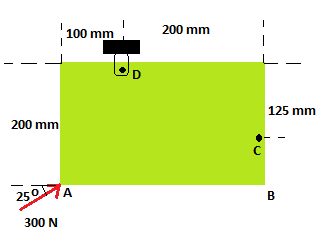# A 300 N force is applied at A as shown. Determine (a) the moment of the 300 N force about D, (b)...

## Question:

A 300 N force is applied at A as shown. Determine (a) the moment of the 300 N force about D, (b) the smallest force applied at B that creates the same moment about D (magnitude and direction).## Moment

The term moment is defined as a measure of force that is able to start rotation in the body when acts on it. The moment is determined as a product of force and the position where the force is applied.

## Answer and Explanation:

Given data:

• The force applied at point A is: {eq}P = 300\;{\rm{N}} {/eq}.
• The angle at which the force is applied is: {eq}\alpha = 25^\circ {/eq}.

(a)

The position vector of point D with respect to point A is,

{eq}\begin{align*} \vec r_{D/A} &= \left( {100\hat i + 200\hat j} \right)\;{\rm{mm}} \\ &= \left( {0.1\hat i + 0.2\hat j} \right)\;{\rm{m}} \\ \end{align*} {/eq}

The expression for the moment vector along the point D is,

{eq}\vec M = \vec r_{D/A} \times P\left( {\cos \theta \hat i + \sin \theta \hat j} \right) {/eq}

Substitute the given values.

{eq}\begin{align*} \vec M &= \left( {0.1\hat i + 0.2\hat j} \right)\;{\rm{m}} \times \left( {300\cos 25^\circ \hat i + 300\sin 25^\circ \hat j} \right)\;{\rm{N}} \\ &= \left( {0.1 \times 300\sin 25^\circ - 0.2 \times 300\cos 25^\circ } \right)\hat k\;{\rm{N}} \cdot {\rm{m}} \\ &= - 41.7\hat k\;{\rm{N}} \cdot {\rm{m}} \\ \end{align*} {/eq}

The magnitude of the moment about D is,

{eq}\begin{align*} M &= \sqrt {\left( { - 41.7\;{\rm{N}} \cdot {\rm{m}}} \right)} \\ &= 41.7\;{\rm{N}} \cdot {\rm{m}} \\ \end{align*} {/eq}

Thus, the moment of force about point D is {eq}41.7\;{\rm{N}} \cdot {\rm{m}} {/eq} along the downward direction.

(b)

The expression for the moment at point B is,

{eq}M = F \times 0.2\;{\rm{m}} {/eq}

Substitute the given values.

{eq}\begin{align*} 41.7\;{\rm{N}} \cdot {\rm{m}} &= F \times 0.2\;{\rm{m}} \\ F &= \dfrac{{41.7\;{\rm{N}} \cdot {\rm{m}}}}{{0.2\;{\rm{m}}}} \\ &= 208.5\;{\rm{N}} \\ \end{align*} {/eq}

Thus, the minimum force at point B that exerts same moment is {eq}208.5\;{\rm{N}} {/eq}.

#### Learn more about this topic:Torque in Physics: Equation, Examples & Problems

from Physics: Middle School

Chapter 3 / Lesson 13
43K